Foxtable(狐表)用户栏目专家坐堂 → 要得到按用户输入的时间条件来得到相应的统计报表，用以下的代码后出错，请指正，谢谢。

共有2440人关注过本帖树形打印复制链接

# 主题：要得到按用户输入的时间条件来得到相应的统计报表，用以下的代码后出错，请指正，谢谢。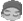soap_lwb
1楼 | 信息 | 搜索 | 邮箱 | 主页 | UC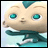加好友发短信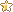下载信息  [文件大小：   下载次数： ]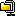点击浏览该文件:管理项目1.zip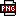此主题相关图片如下：统计结果.png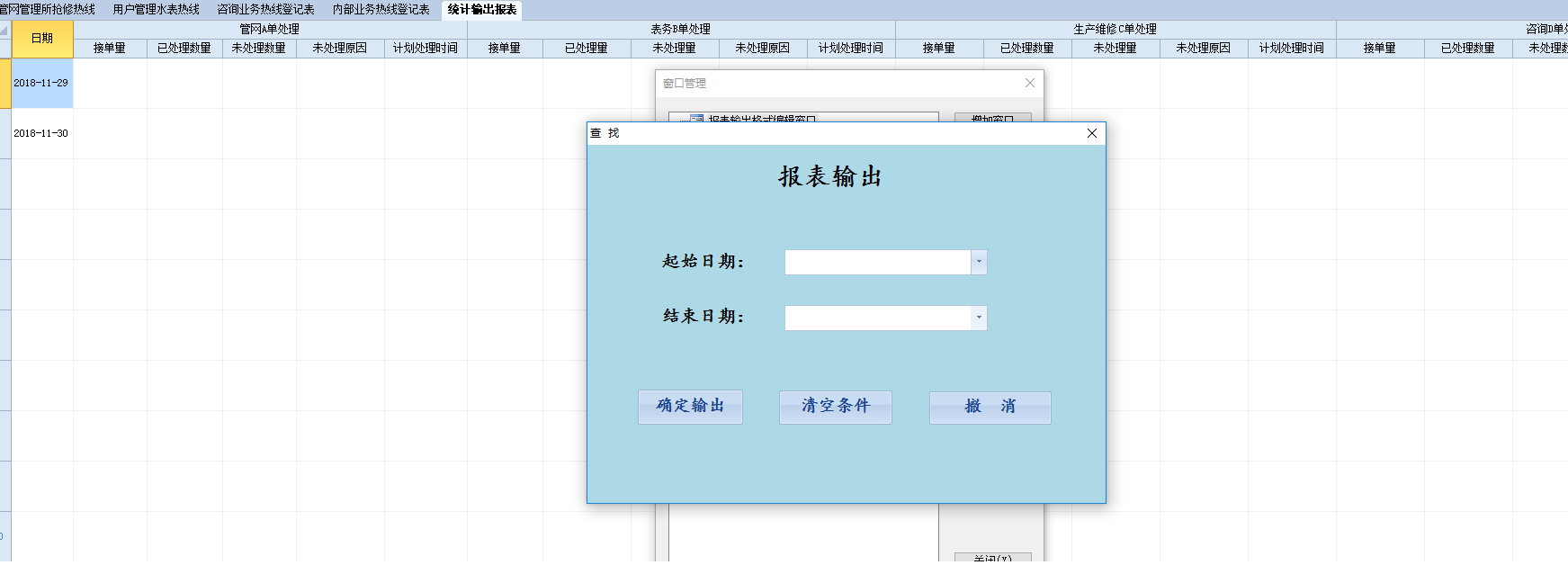Dim tjb As String = "统计表1"Dim b As New SQLGroupTableBuilder(tjb,"管网管理所抢修热线")b.Groups.AddExp("来电时间","CDate(日期)")b.Totals.AddExp("表A_接单量","1")b.Totals.AddExp("表A_已处理数量","iif(是否解决=true,1,0)")b.Totals.AddExp("表A_未处理数量","iif(是否解决=true,0,1)")Dim dd As Date = "2018-10-01"b.filter = "来电时间>=#" & dd & "# and 来电时间 < #" & dd.addmonths(1) & "#"'b.BuildDim d As fxDataSource = b.BuildDataSource()Dim bms As String = "用户管理水表热线|公司内部业务热线登记表|咨询业务热线登记表"For Each bm As String In bms.split("|")    Dim b1 As New SQLGroupTableBuilder(tjb,bm)    b1.Groups.AddExp("来电时间","CDate(日期)")    b1.Totals.AddExp(bm & "_接单量","1")    b1.Totals.AddExp(bm & "_已处理数量","iif(是否解决=true,1,0)")    b1.Totals.AddExp(bm & "_未处理数量","iif(是否解决=true,0,1)")    b.filter = "来电时间>=#" & dd & "# and 来电时间 < #" & dd.addmonths(1) & "#"    'b1.Build    Dim d1 As fxDataSource = b1.BuildDataSource()    d.Combine("来电时间",d1,"来电时间")Nextd.Show(tjb)MainTable = Tables(tjb) '打开生成的统计表"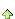2楼 | 信息 | 搜索 | 邮箱 | 主页 | UC加好友发短信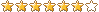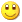Post By：2018/12/3 11:31:00 [只看该作者]3楼 | 信息 | 搜索 | 邮箱 | 主页 | UC加好友发短信Post By：2018/12/3 11:39:00 [只看该作者]

 Dim Filter1 As String = "1=1" With e.Form.Controls("StartDate")    If .Value IsNot Nothing Then        If Filter1 >"" Then            Filter1 = Filter1 & " And "        End If        Filter1 = Filter1 & "来电时间 >= #" & .Value & "#"    End IfEnd WithWith e.Form.Controls("EndDate")    If .Value IsNot Nothing Then        If Filter1 >"" Then            Filter1 = Filter1 & " And "        End If        Filter1 = Filter1 & "来电时间 <= #" & .Value & "#"    End IfEnd With   Dim tjb As String = "统计表1"Dim d As fxDataSourceDim bms As String = "内部业务热线登记表|咨询业务热线登记表|用户管理水表热线|管网管理所抢修热线"Dim i As Integer = 0For Each bm As String In bms.split("|")    Dim b1 As New SQLGroupTableBuilder(tjb,bm)    b1.Groups.AddExp("来电时间","来电时间")    b1.Totals.AddExp(bm & "_接单量","1")    b1.Totals.AddExp(bm & "_已处理数量","iif(是否解决=true,1,0)")    b1.Totals.AddExp(bm & "_未处理数量","iif(是否解决=true,0,1)")    b1.filter = filter1    Dim d1 As fxDataSource = b1.BuildDataSource()    If i = 0 Then        d = d1    Else        d.Combine("来电时间",d1,"来电时间")    End IfNextd.Show(tjb)MainTable = Tables(tjb) '打开生成的统计表"soap_lwb
4楼 | 信息 | 搜索 | 邮箱 | 主页 | UC加好友发短信此主题相关图片如下：统计后结果1203.png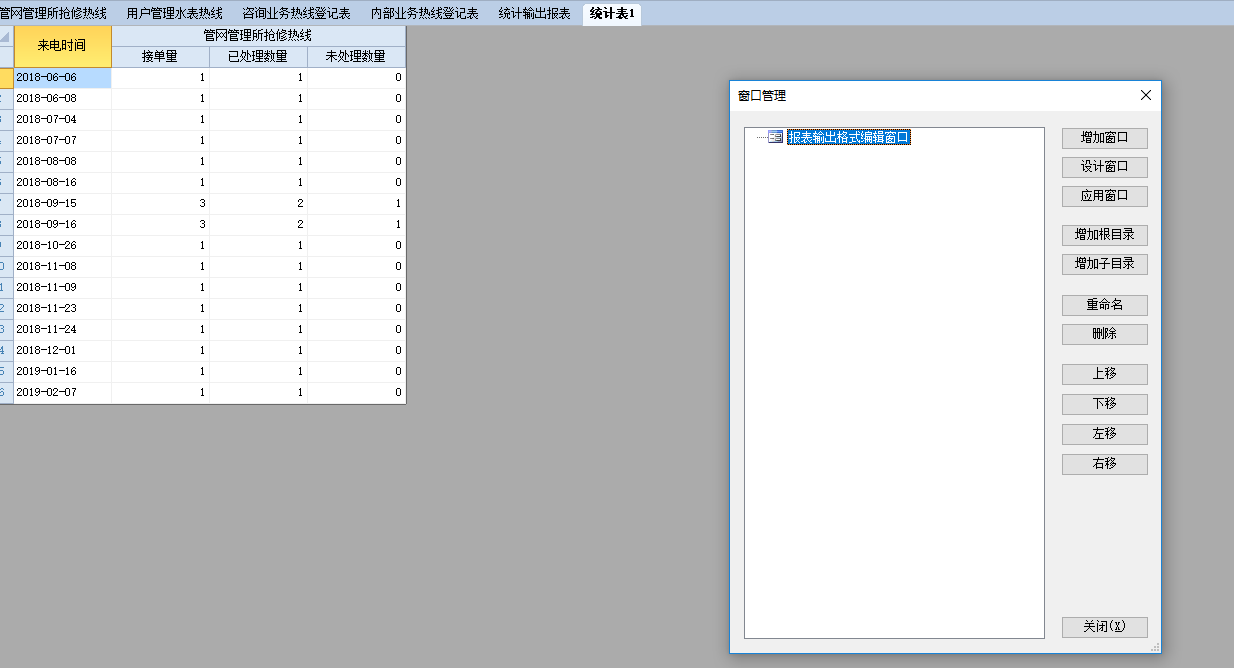5楼 | 信息 | 搜索 | 邮箱 | 主页 | UC加好友发短信Post By：2018/12/3 12:42:00 [只看该作者]

 Dim Filter1 As String = "1=1" With e.Form.Controls("StartDate")    If .Value IsNot Nothing Then        If Filter1 >"" Then            Filter1 = Filter1 & " And "        End If        Filter1 = Filter1 & "来电时间 >= #" & .Value & "#"    End IfEnd WithWith e.Form.Controls("EndDate")    If .Value IsNot Nothing Then        If Filter1 >"" Then            Filter1 = Filter1 & " And "        End If        Filter1 = Filter1 & "来电时间 <= #" & .Value & "#"    End IfEnd With   Dim tjb As String = "统计表1"Dim d As fxDataSourceDim bms As String = "内部业务热线登记表|咨询业务热线登记表|用户管理水表热线|管网管理所抢修热线"Dim i As Integer = 0For Each bm As String In bms.split("|")    Dim b1 As New SQLGroupTableBuilder(tjb,bm)    b1.Groups.AddExp("来电时间","来电时间")    b1.Totals.AddExp(bm & "_接单量","1")    b1.Totals.AddExp(bm & "_已处理数量","iif(是否解决=true,1,0)")    b1.Totals.AddExp(bm & "_未处理数量","iif(是否解决=true,0,1)")    b1.filter = filter1    Dim d1 As fxDataSource = b1.BuildDataSource()    If i = 0 Then        d = d1    Else        d.Combine("来电时间",d1,"来电时间")    End If     i += 1Nextd.Show(tjb)MainTable = Tables(tjb) '打开生成的统计表"soap_lwb
6楼 | 信息 | 搜索 | 邮箱 | 主页 | UC加好友发短信如果在统计表的最后一行需要增加一行汇总统计，如何加代码7楼 | 信息 | 搜索 | 邮箱 | 主页 | UC加好友发短信Post By：2018/12/3 15:25:00 [只看该作者]

 以下是引用soap_lwb在2018/12/3 14:18:00的发言：如果在统计表的最后一行需要增加一行汇总统计，如何加代码   设置汇总模式，或者合计模式   Dim Filter1 As String = "1=1" With e.Form.Controls("StartDate")    If .Value IsNot Nothing Then        If Filter1 >"" Then            Filter1 = Filter1 & " And "        End If        Filter1 = Filter1 & "来电时间 >= #" & .Value & "#"    End IfEnd WithWith e.Form.Controls("EndDate")    If .Value IsNot Nothing Then        If Filter1 >"" Then            Filter1 = Filter1 & " And "        End If        Filter1 = Filter1 & "来电时间 <= #" & .Value & "#"    End IfEnd With   Dim tjb As String = "统计表1"Dim d As fxDataSourceDim bms As String = "内部业务热线登记表|咨询业务热线登记表|用户管理水表热线|管网管理所抢修热线"Dim i As Integer = 0For Each bm As String In bms.split("|")    Dim b1 As New SQLGroupTableBuilder(tjb,bm)    b1.Groups.AddExp("来电时间","来电时间")    b1.Totals.AddExp(bm & "_接单量","1")    b1.Totals.AddExp(bm & "_已处理数量","iif(是否解决=true,1,0)")    b1.Totals.AddExp(bm & "_未处理数量","iif(是否解决=true,0,1)")    b1.filter = filter1    Dim d1 As fxDataSource = b1.BuildDataSource()    If i = 0 Then        d = d1    Else        d.Combine("来电时间",d1,"来电时间")    End If    i += 1Nextd.Show(tjb)MainTable = Tables(tjb) '打开生成的统计表" Dim t As Table = Tables(tjb)Dim g As Subtotalgroupt.SubtotalGroups.Clear()t.GroupAboveData = Falset.TreeVisible = Falset.SpillNode = True g = New Subtotalgroupg.Aggregate = AggregateEnum.Sumg.GroupOn = "*"Dim ls As new List(Of String)For Each c As Col In t.Cols    If c.IsNumeric Then        ls.add(c.Name)    End IfNextg.TotalOn = String.join(",", ls.ToArray)g.Caption = "合计"t.SubtotalGroups.Add(g) t.Subtotal()